Warning

# Segmentation

The assignment deals with image segmentation. The goal is to classify all image pixels to distinct classes, often into foreground and background. The foreground pixels correspond to one or more objects which we are interested in. The background pixels correspond to the remaining part of the image. We will deal with semi-automated segmentation methods. The user is expected to provide certain sample of foreground and background pixels at the beginning, e.g. by drawing a stroke over the foreground and another stroke over the background. The segmentation is computed based on this input.

## Part 1 - pixelwise statistical segmentation

### Overview

We deal with two segmentation methods in the first part of the assignment. The first segmentation method is suitable for grayscale images. The second method deals with color images (namely RGB images). Both methods share two key assumptions:

1. It is assumed that color distribution of the sample foreground pixels provided by the user represents color distribution of all foreground pixels. The same assumption holds for the background pixels.
2. It is assumed that color distributions of the foreground and background pixels are different.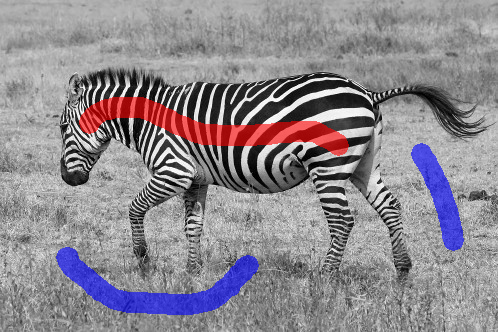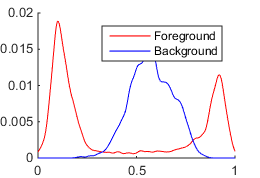Normalized and smoothed histograms of intensities (one for blue background pixels, the other for red foreground pixels) computed from the sample pixels provided by the user by drawing three strokes.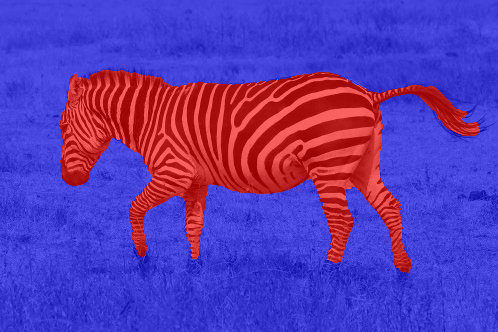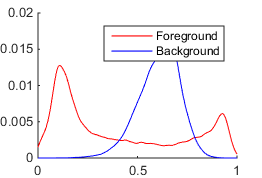Normalized and smoothed histograms of pixels intensities computed from nearly optimal segmentation.

Two probabilistic distributions of color are learned from sample pixels provided by the user: for the foreground and background. Then it is possible to compute the probability of any pixel belonging to the foreground or background. Each pixel is classified to the more likely class. The method suitable for grayscale images models the probability distribution by a normalized histogram. The method suitable for color images uses multivariate Gaussian mixture model (GMM).

### Formalization

The input of the segmentation algorithm is a grayscale or RGB image of size $H \times W$. Each pixel is uniquely determined by a single index $t \in T = \{ 1 \ldots H \cdot W \}$ proceeding in column-major order, as usual in Matlab.

The user is expected to provide a sample of foreground pixels $T_1$ and background pixels $T_2$ such that: $$T_1 \subseteq T, \; T_2 \subseteq T, \; T_1 \cap T_2 = \emptyset$$

Let us denote $x_t$ value of the pixel $t$. For grayscale images, $x_t \in [0, 1]$ is a pixel intensity represented as double. For RGB images, $x_t = (x^R_t, x^G_t, x^B_t) \in [0, 1]^3$ is 3D vector of RGB values.

Based on the set of sample foreground pixels $T_1$ and sample background pixels $T_2$, two probability density functions (PDF) are estimated: $$p_y(x), \; y \in \{1, 2\}$$

The distribution $p_1(x)$ denotes a probability of any foreground pixel having value $x$. The distribution $p_2(x)$ is a probability of any background pixel having value $x$.

The goal of segmentation is to determine $y_t \in \{1, 2\}$ for each pixel $t \in T$, i.e. to decide whether the pixel $t$ belongs to foreground or background. We will choose $y_t$ maximizing the pixel probability over both distributions: $$y_t = \arg \max_{y \in \{1, 2\}} p_y(x_t)$$

In the case that foreground and background probability are equal for some pixel $t \in T$, i.e. $p_1(x_t) = p_2(x_t)$, the pixel class $y_t$ is chosen arbitrarily as $1$ or $2$.

### Histogram based segmentation

The first method is suitable for grayscale images. It models probabilistic distribution functions (PDFs) of intensities for foreground and background pixels by smoothed and normalized histograms.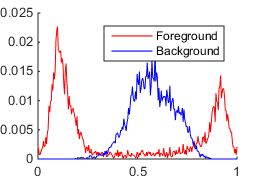Left: The foreground (red) and background (blue) sample pixels are used to estimate PDFs of foreground and background pixels. Middle: The distributions are approximated by smoothed and normalized histograms. Right: The non-smoothed histogram would be too noisy.

The discrete PDFs of foreground $p_1(x)$ and background $p_2(x)$ pixel intensities are constructed as follows:

1. Use the provided sample foreground pixels $T_1$ to compute a histogram of intensities, using the specified number of bins. The bin centers should be spaced uniformly and span the interval $[0, 1]$. Use Matlab function hist(x, xbins) to compute the histogram. Do the same for sample background pixels $T_2$.
2. Smooth the histograms by convolving them with Gaussian lowpass filter. Use Matlab function fspecial('gaussian', [1 filter_size], sigma) to generate the filter. Choose filter_size as the smallest odd integer bigger or equal to 5*sigma. Use Matlab function conv(u, v, 'same') to compute the convolution.
3. Normalize the histograms in order to get valid PDFs.

Once the PDFs are estimated, the probability $p_y(x_t)$ of pixel $t \in T$ having the intensity $x_t \in [0, 1]$ is given by the bin corresponding to $x_t$ in the normalized and smoothed histogram. The pixels are classified to maximize the probability: $$y_t = \arg \max_{y \in \{1, 2\}} p_y(x_t)$$

Download the package containing source codes and testing images. It will be used in both parts of the assignment.

1. Implement the following functions:
• pdf = hist_pdf(x, num_bins, sigma): Create the histogram of input data samples having the specified number of bins, smooth by the convolution with Gaussian and normalize to a valid PDF.
• prob = hist_prob(x, pdf): Compute probabilities of the input data sample in the specified discrete PDF.
• lab_out = segmentation_hist(img, lab_in, num_bins, sigma): Segment the grayscale image based on various statistical properties of the foreground and background pixel intensities. The probability distributions of pixel intensities are modeled using smoothed and normalized histograms. The histograms are constructed from the initial partial labeling.
2. Test your implementation using the script hw_segmentation_hist included in the package. To segment your own images and to define custom initial labeling, use simple GUI implemented in the function segmentation_gui(img_path).

### Gaussian mixture model based segmentation

It would be possible to use 3D histogram to represent probability distribution of RGB colors. However, if we had one bin for each of possible $256^3$ RGB colors (for 8-bit image), the majority of bins would be empty. Another option would be to use lower number of bins or partition the $[0, 1]^3$ RGB cube non-uniformly, e.g. using k-d trees. We will use continuous distribution called Gaussian mixture model (GMM) instead.

The $D$-dimensional GMM with $K$ components is defined as: $$\sum_{k=1}^K \phi_k \; \mathcal{N}(x; \mu_k, \Sigma_k) = \sum_{k=1}^K \phi_k \; (2\pi)^{-D/2} \; |\Sigma_k|^{-1/2} \; \exp \left( -\frac{1}{2} (x - \mu_k)^T \Sigma_k^{-1} (x - \mu_k) \right)$$ It is a weighted summation of $K$ multivariate normal distributions $\mathcal{N}(x; \mu_k, \Sigma_k)$ in $D$ dimensions. The prior probability of $k$-th normal distribution is denoted by $\phi_k \in [0, 1]$. It holds $\sum_{k=1}^K \phi_k = 1$. Each normal distribution is determined by its mean vector $\mu_k \in \mathbb{R}^D$ and covariance matrix $\Sigma_k \in \mathbb{R}^{D \times D}$. $|\Sigma_k|$ denotes determinant of the covariance matrix, $\Sigma_k^{-1}$ its inverse and $(x - \mu_k)^T$ is transposed vector.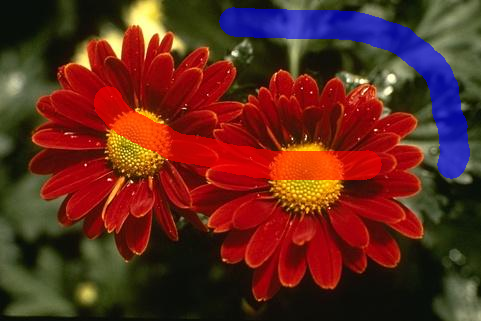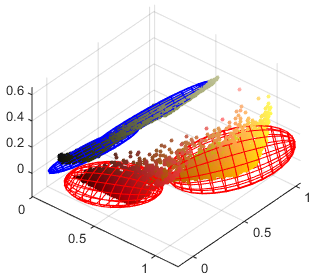The foreground (red) and background (blue) sample pixels are used to estimate PDFs of foreground and background pixels modeled by GMMs. Individual components of GMM are formed by multivariate normal distributions. Both foreground and background GMMs are formed by two normal distributions in this case. The normal distributions are plotted as ellipsoids. The ellipsoid center corresponds to the mean vector and its shape to the covariance matrix of the respective normal distribution.

We will use two GMMs to represent probabilities of the pixel color $x_t \in [0, 1]^3$ in foreground ($y = 1$) and background ($y = 2$): $$p_y(x_t) = p(x_t; \theta_y) = \sum_{k=1}^K \phi_{y,k} \; \mathcal{N}(x_t; \mu_{y,k}, \Sigma_{y,k})$$

Both foreground and background GMM is fully specified by a set of its parameters: $$\theta_y = \{ \phi_{y,1} \ldots \phi_{y,K}, \mu_{y,1} \ldots \mu_{y,K}, \Sigma_{y,1} \ldots \Sigma_{y,K} \}$$

The goal is to use the sample foreground pixels $T_1$ to determine the parameters $\theta_1$ and the sample background pixels $T_2$ to determine $\theta_2$. We will describe estimation of $\theta_1$ from $T_1$. Parameters $\theta_2$ are estimated analogously.

Let us assume that RGB color $x_t \in [0, 1]^3$ of the pixel $t \in T_1$ was generated by foreground GMM. It means that it was generated by one of $K$ multivariate normal distributions forming GMM. Let us denote a hidden variable $c_t \in \{1 \ldots K\}$ telling which component generated $x_t$. It holds: \begin{align*} p(x_t; \theta_1) &= \sum_{c=1}^K p(x_t, c; \theta_1)\\ &= \sum_{c=1}^K p(x_t \mid c; \theta_1) \; p(c; \theta_1)\\ &= \sum_{c=1}^K \mathcal{N}(x_t; \mu_{1,c}, \Sigma_{1,c}) \; \phi_{1,c} \end{align*}

We split estimation of $\theta_1$ into two steps. In the first step, we estimate $c_t \in \{1 \ldots K\}$ for each pixel $t \in T_1$. This is done by splitting colors of pixels from $T_1$ into $K$ clusters. The identifier of the cluster containing color $x_t$ is assigned to $c_t$. The clustering is performed by k-means algorithm. The algorithm is available in Matlab: idx = kmeans(x, k, 'MaxIter', kmeans_iter).

In the second step, pixels in each cluster are used to estimate parameters of the corresponding multivariate normal distribution and its prior probability. Let us denote $C_{1,k}$ a set of all pixels from $T_1$ which were assigned to cluster $k$ by k-means algorithm: $$C_{1,k} = \{ t \mid t \in T_1, c_t = k \}$$

The parameters $\theta_1$ are then found by maximum likelihood estimation (MLE): \begin{align*} \phi_{1,k} &= \frac{|C_{1,k}|}{|T_1|}\\ \mu_{1,k} &= \frac{1}{|C_{1,k}|} \sum_{t \in C_{1,k}} x_t\\ \Sigma_{1,k} &= \frac{1}{|C_{1,k}|} \sum_{t \in C_{1,k}} (x_t - \mu_{1,k})^T (x_t - \mu_{1,k})\\ \end{align*}

I.e. $\phi_{1,k}$ is equal to the relative size of the $k$-th cluster, $\mu_{1,k}$ is the mean color of pixels assigned to the $k$-th cluster and $\Sigma_{1,k}$ is their covariance. The covariance $\Sigma_{1,k}$ can be estimated by Matlab function cov(x, 1), assuming that individual pixels form rows of the matrix x. The second parameter tells that we want to obtain maximum likelihood estimate. Calling cov(x, 0) or cov(x) returns the best unbiased estimate, in which the summation is normalized by dividing it with $|C_{1,k}| - 1$ instead of $|C_{1,k}|$.

Once both GMMs are estimated, we classify each pixel $t$ according to its color $x_t$ to the class $y_t$ maximizing its probability: \begin{align*} y_t &= \arg \max_{y \in \{1, 2\}} p(x_t; \theta_y)\\ &= \arg \max_{y \in \{1, 2\}} \sum_{k=1}^K \phi_{y,k} \; \mathcal{N}(x_t; \mu_{y,k}, \Sigma_{y,k})\\ \end{align*}

1. Implement the following functions:
• [priors, means, covs] = gmm_estimation(x, c): Estimate Gaussian mixture model (GMM) by maximizing the likelihood. The input is formed by sample vectors split to clusters. Each cluster corresponds to one GMM component. The output is formed by estimated prior probabilities, mean vectors and covariance matrices for all components.
• prob = gmm_prob(x, priors, means, covs): Compute probabilities of the specified samples. The probability distribution is GMM specified by its prior probabilities, mean vectors and covariance matrices of individual components.
• lab_out = segmentation_gmm(img, lab_in, num_comps, kmeans_iter): Segment the RGB image based on various statistical properties of the foreground and background pixel colors. The probability distributions of RGB colors are modeled using mixture of Gaussians (GMM). The GMMs are constructed from the initial partial labeling.
2. Test your implementation using the script hw_segmentation_gmm included in the package. To segment your own images and to define custom initial labeling, use simple GUI implemented in the function segmentation_gui(img_path).

## Part 2 - ensuring smoothness

### Overview

We dealt with statistical segmentation of images in the first part of the assignment. The main drawback was that we were classifying each pixel independently on its neighboring pixels. The classification was based purely on the pixel color probability in the estimated foreground and background PDF. This approach usually leads to non-smooth segmentation with many small areas of incorrectly classified pixels, i.e. foreground pixels whose color is more probable in the estimated background PDF than in the estimated foreground PDF, or vice versa for background pixels.

In the second part of the assignment, we will introduce a method which encourages the neighboring pixels to be classified to the same class. Then if there is some foreground pixel whose color is more likely background, but its neighboring pixel colors are more likely foreground, the pixel is classified correctly as foreground.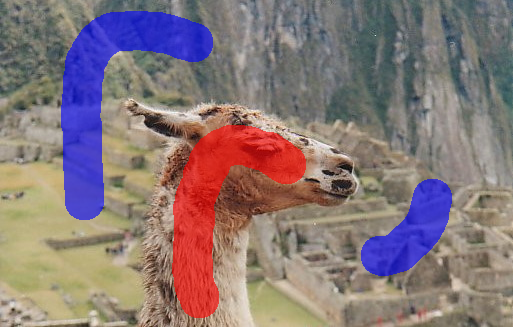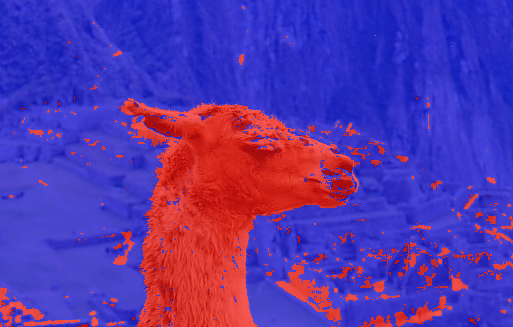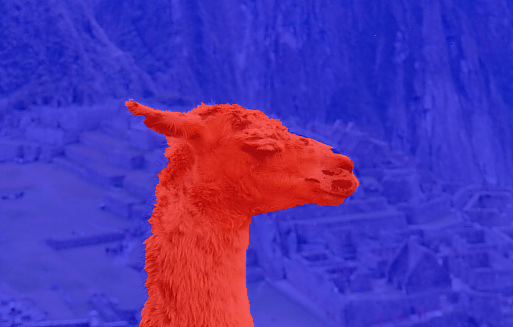Left: The input image and its labeling provided by user. Middle: Statistical GMM based segmentation classifies each pixel independently and results in non-smooth segmentation with many small groups of incorrectly classified pixels. Right: Graph cut based segmentation takes neighborhood of pixels into an account and results in smooth segmentation.

### Cost minimization

In the first part of the assignment, we classified each pixel $t \in T$ independently in order to maximize probability of its RBG color $x_t \in [0, 1]^3$ over the estimated foreground GMM of pixel colors $p(x; \theta_1)$ and the background GMM $p(x; \theta_2)$: \begin{align*} y_t &= \arg \max_{y \in \{1, 2\}} p(x_t; \theta_y)\\ &= \arg \max_{y \in \{1, 2\}} \log p(x_t; \theta_y)\\ &= \arg \min_{y \in \{1, 2\}} (- \log p(x_t; \theta_y) )\\ \end{align*}

The second row follows from the fact that $\log$ is a strictly increasing function and thus $y$ maximizing $p(x_t; \theta_y)$ also maximizes $\log p(x_t; \theta_y)$. The third row follows from the fact that maximization over values is equivalent to minimization over negative values.

The first improvement over the first part is ensuring that pixels marked by the user as foreground ($t \in T_1$) remain foreground in the final segmentation ($y_t = 1$). The same should hold for pixels marked as background (set $T_2$). The classification problem can be reformulated like this: $$y_t = \begin{cases} 1, \hspace{123pt} t \in T_1\\ 2, \hspace{123pt} t \in T_2\\ \arg \min_{y \in \{1, 2\}} (- \log p(x_t; \theta_y) ), \hspace{5pt} t \in T \setminus (T_1 \cup T_2)\\ \end{cases}$$

Let us introduce the following cost $q_y(t)$ for each class $y \in \{1,2\}$ (foreground and background) and each pixel $t \in T$. The cost of assigning initially foreground pixel to the background class is set to $\infty$ which ensures that such pixel is always classified as foreground. The pixels marked by the user as background are treated similarly. \begin{align*} q_1(t) =& \begin{cases} - \log p(x_t; \theta_1), \hspace{5pt} t \in T \setminus T_2\\ \infty, \hspace{54pt} t \in T_2\\ \end{cases}\\ q_2(t) =& \begin{cases} - \log p(x_t; \theta_2), \hspace{5pt} t \in T \setminus T_1\\ \infty, \hspace{54pt} t \in T_1\\ \end{cases}\\ \end{align*}

The pixels are classified in order to minimize the cost $q_y(t)$: $$y_t = \arg \min_{y \in \{1, 2\}} q_y(t)$$

### Introducing graph cuts

We will show how to formulate the problem of minimizing $q_y(t)$ for each $t \in T$ in the framework of finding maximum flow in the specifically constructed undirected graph. The problem is equivalent to the problem of finding minimum cut in the same graph.

Let us assume that we have been given $2 \times 2$ image. Let us denote each pixel by single index $T = \{1, 2, 3, 4\}$, as it is usual in Matlab. Let us further assume that we have been given the costs $q_y(t)$ for each $y \in \{1, 2\}$ and $t \in T$. Let us assume that: $$q_1(1) < q_2(1) \Rightarrow y_1 = 1 \qquad q_1(3) < q_2(3) \Rightarrow y_3 = 1\\ q_1(2) < q_2(2) \Rightarrow y_2 = 1 \qquad q_1(4) > q_2(4) \Rightarrow y_4 = 2\\$$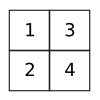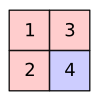Left: Example input image of size $2 \times 2$ with pixels $T = \{1, 2, 3, 4\}$. Right: Hypothetically optimal segmentation of the input image with pixels $1, 2, 3$ segmented as foreground and $4$ as background.

Let us now construct an undirected graph $G = (V, E)$. It contains one vertex $v_t$ for each pixel $t \in T$, special vertex $w_1$ for foreground class and special vertex $w_2$ for background class. There is the undirected edge connecting each pixel vertex $v_t$ both to the foreground vertex $w_1$ and the background vertex $w_2$. Each undirected edge $\{w_y, v_t\}$ is assigned a weight equal to the cost $q_y(t)$. \begin{align*} V &= \{w_1, w_2\} \cup \{v_t \mid t \in T\}\\ E &= \{ \{w_y, v_t\} \mid y \in \{1,2\}, t \in T\}\\ \mathrm{weight}(\{w_y, v_t\}) &= q_y(t)\\ \end{align*}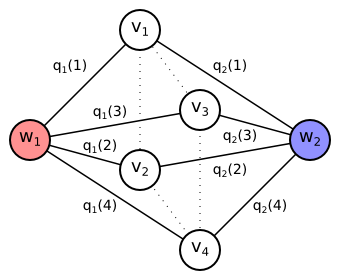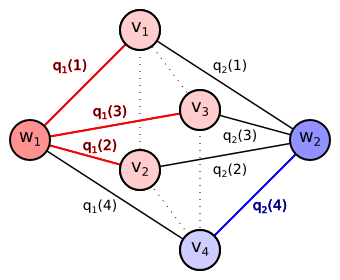Left: The undirected graph constructed for sample $2 \times 2$ image. It contains one vertex $v_t$ for each pixel $t \in T$, foreground vertex $w_1$ and background vertex $w_2$. Each pixel vertex $v_t$ is connected to each special vertex $w_y$ with the undirected edge having assigned the cost $q_y(t)$. Right: Minimum cut separating the foreground vertex $w_1$ from the background vertex $w_2$ (imagine the red and blue edges removed) corresponds to the optimal segmentation. Size of the cut is $\color{red}{q_1(1)} + \color{red}{q_1(2)} + \color{red}{q_1(3)} + \color{blue}{q_2(4)}$.

The problem of finding minimum cut reposes in finding subset of edges $C^* \subseteq E$ separating the source foreground vertex $w_1$ from the sink background vertex $w_2$ such that sum of weights of the edges included in the cut is minimum: $$C^* = \arg \min_{C \subseteq E} \left\{ \sum_{\{w_y,v_t\} \in C} q_y(t) \mid \text{ there is no path from } w_1 \text{ to } w_2 \text{ in } (V, E \setminus C) \right\}$$

It is obvious that for our graph every minimum cut must contain either the edge $\{w_1,v_t\}$ or the edge $\{w_2,v_t\}$ for every pixel $t \in T$. If it contained none of them, there would still exist the path from $w_1$ to $w_2$ over $v_t$ after removing the cut edges. If it contained both of them, the cut would not be minimum, since it is sufficient to remove one of the edges $\{w_1,v_t\}$, $\{w_2,v_t\}$ for each $t \in T$ to separate $w_1$ from $w_2$. The minimum cut will therefore for each pixel contain the edge with the lower cost: $$C^* = \left\{ \{w_y, v_t\} \mid t \in T, y = \arg \min_{y' \in \{1,2\}} q_{y'}(t) \right\}$$

We will now extend the proposed undirected graph by adding more edges. Our goal is to prefer the neighboring vertices to be classified to the same class if possible. We will add an undirected edge between every pair of pixels $t, s \in T$ which are neighbors. Let us denote the set of all neighboring pixel pairs as $P$, i.e. $\{t, s\} \in P$. We will define the set of all neighboring pixels $P$ later. The edge connecting two neighboring pixel vertices is assigned a certain weight $r(t,s)$. Since the edge is undirected, the weight can be also denoted $r(s,t)$, i.e. the function $r$ is symmetric in its arguments. \begin{align*} V &= \{w_1,w_2\} \cup \{v_t \mid t \in T\}\\ E &= \{ \{w_y,v_t\} \mid y \in \{1,2\}, t \in T \} \cup \{ \{v_t,v_s\} \mid \{t,s\} \in P \}\\ \mathrm{weight}(\{w_y,v_t\}) &= q_y(t)\\ \mathrm{weight}(\{v_t,v_s\}) &= r(t,s) = r(s,t)\\ \end{align*}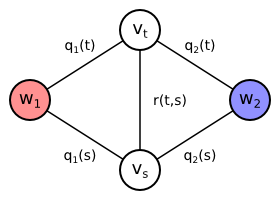Extended graph containing also edge $\{v_t,v_s\}$ between two neighboring pixel vertices. The edge is assigned the weight $r(t,s)$ depending on pixel colors.

Let us assume that pixels $t$ and $s$ are neighbors, i.e. $\{t,s\} \in P$. The edge $\{v_t,v_s\}$ is therefore included in the graph. Then every minimum cut assigning $t$ and $s$ to different classes must also contain the edge $\{v_t,v_s\}$. Otherwise there would be a path from $w_1$ to $w_2$ not using any edges contained in the cut: $(w_1,v_t,v_s,w_2)$ or $(w_1,v_s,v_t,w_2)$. It means that if the minimum cut assigns the pixels $t$ and $s$ to different classes, it also includes the extra weight $r(t,s)$. The consequence is that two neighboring pixels are only classified differently if it is profitable to pay the extra cost $r(t,s)$.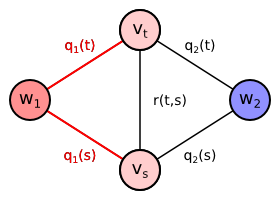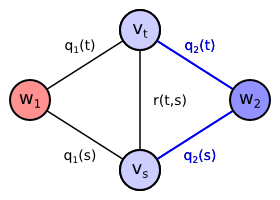Cost: $\color{red}{q_1(t)} + \color{red}{q_1(s)}$ Cost: $\color{blue}{q_2(t)} + \color{blue}{q_2(s)}$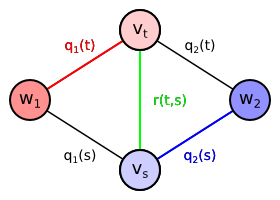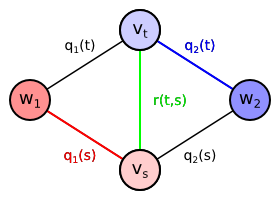Cost: $\color{red}{q_1(t)} + \color{blue}{q_2(s)} + \color{green}{r(t,s)}$ Cost: $\color{blue}{q_2(t)} + \color{red}{q_1(s)} + \color{green}{r(t,s)}$ Possible minimum cuts separating the foreground vertex $w_1$ from the background vertex $w_2$. Each cut determines unique segmentation of pixels $t, s$. The cut with the minimum cost determines the optimum segmentation. Top: If both pixels are segmented to the same class, the minimum cut only uses edges connecting pixel vertices with foreground or background vertices (imagine red and blue edges removed). Bottom: If each pixel is classified differently, the edge $\{v_s,v_t\}$ must be also part of the minimum cut. Otherwise there would still exist a path from $w_1$ to $w_2$ after the cut removal (imagine only red and blue edges removed, black and green edge kept).

There are two possible ways how to determine set $P$ containing pairs of neighboring pixels. We can use so called 4-neighborhood where all vertically and horizontally adjacent pixels are considered neighbors, i.e. every pixel (except the boundary ones) has 4 neighbors (left, right, top, bottom). We can also use 8-neighborhood where also diagonally adjacent pixels are considered neighbors, i.e. every pixel (except the boundary ones) has 8 neighbors.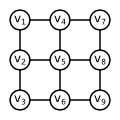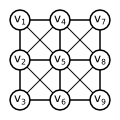Comparison of 4-neighborhood and 8-neighborhood for a hypothetical $3 \times 3$ input image.

It remains to define the costs $r(t,s)$ of the undirected edges $\{v_t,v_s\}$ connecting pairs of neighboring pixels $\{t,s\} \in P$. Remember that the edge $\{v_t,v_s\}$ is only included in the cut if the pixels $t$ and $s$ are classified differently (one as foreground, the other as background). Therefore $r(t,s)$ should express price paid for violating smoothness of the segmentation.

The main criterion is similarity of pixel RGB colors $x_t, x_s \in [0,1]^3$. If the colors are similar, we strongly prefer both pixels $t$ and $s$ to be classified to the same class. Therefore we set $r(t,s)$ higher for visually similar neighboring pixels. The additional criterion is spatial distance of pixels in the image grid. The horizontally and vertically neighboring pixels are closer than diagonal neighbors. We care more about the closer pixels to be classified identically. Therefore we set $r(t,s)$ higher for the vertical and horizontal neighbors than for diagonal neighbors.

Formally, for each $\{t,s\} \in P$ we compute the following cost: $$r(t,s) = \lambda_1 + \frac{\lambda_2}{d(t,s)} \; \exp \left( - \frac{\|x_t-x_s\|^2}{2 \beta} \right)$$

The parameter $\lambda_1$ scales the constant part of the weight $r(t,s)$. The constant part is usually called Ising prior. The parameter $\lambda_2$ scales the part dependent on $t$ and $s$. The recommended values are $\lambda_1 = 5$ and $\lambda_2 = 45$. Using higher values of $\lambda_1$ and $\lambda_2$ encourages smoother segmentation. Lower values prefer pixel-wise segmentation based on the estimated foreground and background color model.

The term $d(t,s)$ denotes Euclidean distance of pixels in the image grid. It is equal to $1$ for horizontally and vertically neighboring pixels. It is equal to $\sqrt{2}$ for diagonal neighbors.

The term $\|x_t-x_s\|^2$ denotes squared Euclidean distance of pixel colors. The pixel color $x_t \in [0, 1]^3$ is 3D vector of RGB components. $$\|x_t-x_s\|^2 = (x_t^R - x_s^R)^2 + (x_t^G - x_s^G)^2 + (x_t^B - x_s^B)^2$$

The term $\beta$ normalizes Euclidean distance of pixel colors. It is equal to the expected squared Euclidean distance of pixel colors over all image, i.e. over each pair of neighbors $\{t', s'\} \in P$: $$\beta = \frac{1}{|P|} \sum_{\{t',s'\} \in P} \|x_{t'}-x_{s'}\|^2$$

### Implementation

1. Get familiar with the function lab = graphcut(cost_unary, pairs, cost_pair) contained in the package.
• Run the script hw_segmentation_mex which tests whether the provided function graphcut is working on your computer.
• The function graphcut is using library Bk_matlab written in C++ and compiled to MEX file. We provide already compiled binaries for 64-bit versions of Windows, Linux and OS X. For Windows, we provide also alternative binary placed in the directory Bk_matlab\bin\mexw64_alt. If the original binary does not work for you, try to replace it with this one. It may also happen that you need to compile the library for your operating system. The library should be compiled automatically while calling the function graphcut. You only need to set up external compiler in Matlab.
• The function graphcut assumes that the specified graph is undirected, i.e. the matrix pairs contains for each pair of neighbors $\{t,s\} \in P$ either the column [t;s] or the column [s;t], never both of them.
2. Implement the following functions:
• cost = cost_unary(rgb, lab_in, priors1, means1, covs1, priors2, means2, covs2): Compute unary costs for the given RGB values using two estimated GMMs determined by their priors, means and covariances. It should hold $\mathtt{cost(y,t)} = q_y(t)$ for $y \in \{1,2\}$ and $t \in \{1 \ldots \mathtt{size(rgb,2)} \}$.
• [pairs, dists] = build_neighborhood(h, w, neighborhood_type): Build 4-connected or 8-connected neighborhood of pixels and compute their spatial distances. The pairs of neighboring pixels are determined by pairs of their 1D indices in the image grid. Each pair of indices should be included only once, independently on their order. If $\{t,s\} \in P$ are neighbors then pairs should include either the column [t;s] or the column [s;t], never both of them. The vertically and horizontally neighboring pixels have distance $1$, the diagonal neighbors $\sqrt{2}$.
• cost = cost_pair(rgb, pairs, pair_dists, lambda1, lambda2): Compute pairwise costs for pairs of pixels. Each pair is determined by a pair of 1D indices in the image grid. It should hold $\mathtt{cost(i)} = r(t,s) = r(s,t)$ where pairs(:,i) includes either [t;s] or [s;t].
• lab_out = segmentation_graphcut(img, lab_in, num_comps, kmeans_iter, neighborhood_type, lambda1, lambda2): Segment the RGB image using the graphcut algorithm. The probability distributions (GMMs) of background and foreground colors are constructed from the partial input labeling.
3. Test your implementation using the script hw_segmentation_graphcut included in the package. To segment your own images and to define custom initial labeling, use simple GUI implemented in the function segmentation_gui(img_path).

## State-of-the-art instance segmentation methods

Papers:

Implementations: